﻿ Boffin Access Limited - Open Access Journals - Scholarly Publishing-STEM# ADVANCES IN COMPUTER SCIENCES (ISSN:2517-5718)

### Bayo H Lawal*

Department of Statistics & Mathematical Sciences, Kwara State University, Malete, Kwara State, Nigeria

### CitationCitation COPIED

Lawal BH. On Modeling the Double and Multiplicative Binomial Models as Log-Linear Models. Adv Comput Sci.2018 Jan; 1(1):103.

### Abstract

In this paper we have fitted the double binomial and multiplicative binomial distributions as log-linear models using sufficient statistics. This approach is not new as several authors have employed this approach, most especially in the analysis of the Human sex ratio in . However, obtaining the estimated parameters of the distributions may be problematic, especially for the double binomial where the parameter estimate of π may not be readily available from the Log-Linear (LL) parameter estimates. Other issues associated with the LL approach is its implementation in the generalized linear model with covariates. The LL uses far more parameters than the procedure that employs conditional log-likelihoods functions where the marginal likelihood functions are minimized over the parameter space. This is the procedure employed in SAS PROC NLMIXED. The two procedures are essentially equivalent for frequency data. For models with covariates, the LL uses far more parameters and the marginal likelihood functions approach are employed here on three data set having covariates.

### Keywords

Double Binomial; Multiplicative Binomial; Log-Linear; Marginal Likelihood Functions

### Introduction

In the formulations of the multiplicative binomial distribution, in Altham and its corresponding double binomial distribution in Efron, both distributions were characterized with intractable normalizing constants c(n, ψ) and c(n, π) respectively [2,3]. Consequently, these models were implemented in by utilizing a generalized linear model with a Poisson distribution and log link to the frequency data. This approach has earlier being similarly employed in [1,4]. This approach which employs joint sufficient statistics in both distributions was earlier proposed in Lindley & Mersch .

Both distributions are fitted using a Poisson regression model having sufficient statistics from both distributions as explanatory variables with the frequencies being the mean dependent variables. For the Double binomial model (DBM), the sufficient statistics are y log(y) and (n − y) log(n − y). Similar joint sufficient statistics for the multiplicative binomial model (MBM) are y and y(n − y) with the offset being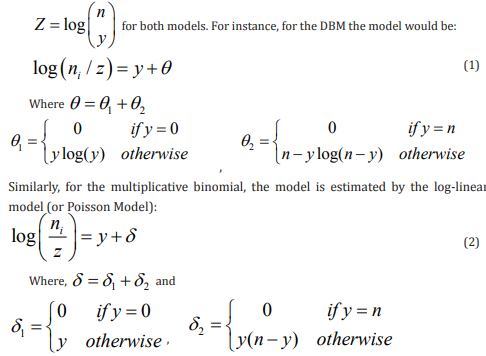However, in recent times, both models have been fully formulated with the intractable normalizing constants fully formulated. These distributions are described later in this paper with the normalizing constants fully formulated. In this study, we compare fitting these two probability models to two example frequency data, two data set examples arising from teratology studies, and randomized complete block design example having binary outcome by the method of sufficient statistics described above and by the method of numerically maximizing the marginal likelihood function arising from engaging the problem as a mixed generalized linear model. The SAS PROC NLMIXED which performs the Maximum Likelihood estimation numerically by using the Adaptive Gaussian Quadrature and Newton-Raphson optimization algorithm. We shall designated the sufficient StatisticsPoisson regression approach as LL, while the marginal likelihood function maximization via PROCNLMIXED is designated MgL in this study. The sufficient statistics procedure uses a Poisson regression with an offset and is implemented in SAS PROC GENMOD.

### Models Under Consideration

We describe in the following section, the two probability distribution models employed in this paper.

The Multiplicative Binomial Model-MBM

[2,6,7] Lovinson proposed an alternative form of the twoparameter exponential family generalization of the binomial distribution first introduced by  which itself was based on the original Cox representation as: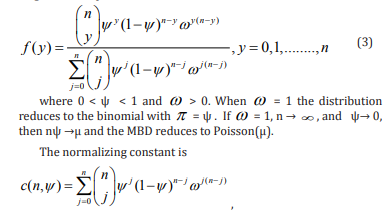the denominator expression in (3) in this case.  presented an elegant characteristics of the multiplicative binomial distribution including its four central moments. His treatment includes generation of random data from the distribution as well as the likelihood profiles and several examples-some of which are similarly employed in this presentation. Following  the probability $\pi$ of success for the Bernoulli trial, that is, P(Y = 1) can be computed from the following expression in (4) as: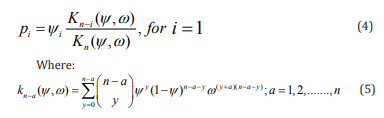with p defined as in (4),  Ψ therefore can be defined as the probability of success weighted by the intra-units association measure  ω which measures the dependence among the binary responses of the n units. Thus if ω = 1, then p =  Ψ and we have independence among the units. However, if ω  = 1, then, p =  Ψ and the units are not independent.

The mean and variance of the LMPD are given respectively as: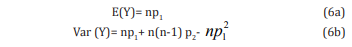### The Double Binomial (DBM) Model

In Feirer et al. , the double binomial distribution was presented, having the pdf form: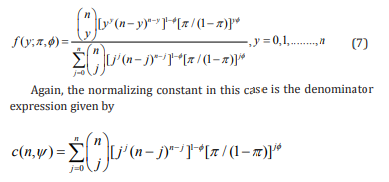### Applications

We apply the models discussed above to two frequency data and to teratology data sets having four and two treatment groups. We first present the analyses for the two frequency data sets in Tables 1 through 5. The estimation of the parameters under each model for the MgL approach uses SAS PROC NLMIXED, using the following log-likelihoods for the MBM (LL1) and DBM (LL2) respectively. The procedure was discussed earlier in the paper.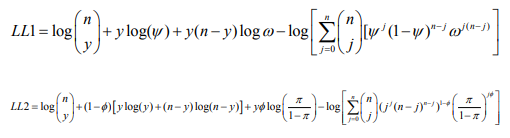Example Data Set I-Geissler Data

The Distribution of males in 6115 families with 12 children in Saxony, previously analyzed in Sokal & Rohlf  is presented in Table 1. The data is originally from Geissler  and had similarly been analyzed in . Here Y ~ binomial (12,π] ). The frequencies are presented as counts having a total sum of 6115. The observed mean for the data is Y_= 6.2306 and the corresponding variance is S2 = 3.4898. Under the binomial model, the estimated mean is 6.2304 and estimated variance being 2.9956. Hence the estimated dispersion parameter DP= Y_ /S2= 2.07 indicating over-dispersion in the data. The estimated probability of occurrence under the binomial model is π = 0.5192. The binomial does not fit the data(X2  = 110.5051 on 11 d.f., p-value=0.0000) because the variance of the data is grossly under estimated by the model. The results of the application of the double binomial and the multiplicative models to this data are presented in Table 2. Further, the mixed model approach is based on one more degree of freedom as it estimates one parameter less than the LL model approach. The Mixed model approach gives the parameter estimates of the distribution. We can obtain the equivalent parameters estimates from the Log-linear (LL) approach for the multiplicative models as follows: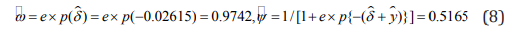For the DB,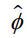can equivalently be obtained as 1 −= 1 − 0.140205 = 0.8598, but the estimated probability  π_ seems intractable in this case and no equivalent solution is available in this case. We may note here that the estimate ψ _ = 0.5165 under the multiplicative model is not an estimate of the success probabilityπ . For this data, we must use the expressions in (4) and (5) to obtain this estimate. Here,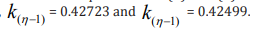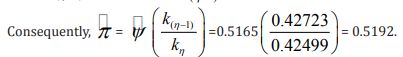The mean and Variance can therefore be computed respectively from (6a) and (6b). Alternatively, the means and variances can be empirically obtained from the fitted models using the elementary principles of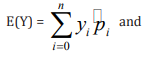In the above Table, Some of the columns are self explanatory. The columns labeled V1 and V2 are cumulative values of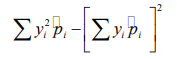for both models respectively. Thus, the mean is the value at y = 12. The variance for the DBM for instance, is computed as 42.3116− (6.2306)2 = 3.4915.

In Table 4 are presented the expected values under both models for the two approaches (LL & MgL), both approaches give exact results as expected. The Table also displays the mean of the distributions under both approaches as well as the empirical variances, designated here as var. We recall that for the observed data in Table 1, y = 6.2306 and s2 = 3.4898.We see from Table 4, that while the two models estimate the mean of the data well, the estimated variance under the binomial model of 12(0.5192)(1−0.5192) = 2.9956 underestimates the observed variance of the data, and this explains the poor fit to the data by the binomial model. On the other hand, for the two models, the variance of the observed data are reasonably well estimated, because of the extra parameter in the model (dispersion parameter) of φ and ω for the DBM and MBM respectively.

Table 4 also displays the corresponding Pearson‘s X2 and the corresponding degrees of freedom (d.f.). Clearly, for this data set, both the double binomial and the multiplicative models fit the data well with the Double binomial being slightly providing a better fit. Although the expected values generated are the same for both fitting approaches, we see that the marginal likelihood (MgL) approach gives a more parsimonious model because it is based on one more degree of freedom.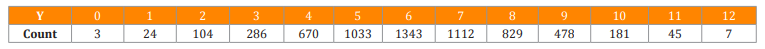Table 1: Distribution of Males in 6115 families with 12 children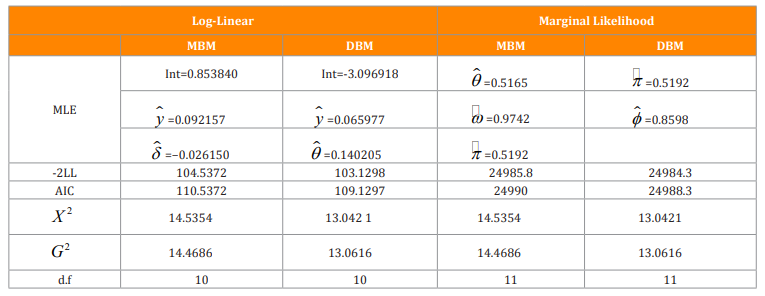Table 2: Parameter estimates under the five Models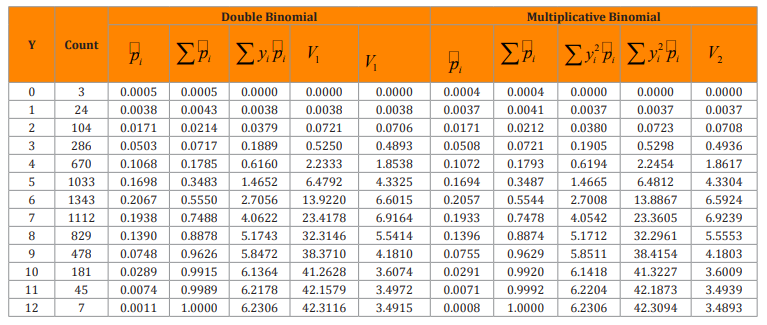Table 3: Empirical Means and Variances for both the DBM and MBM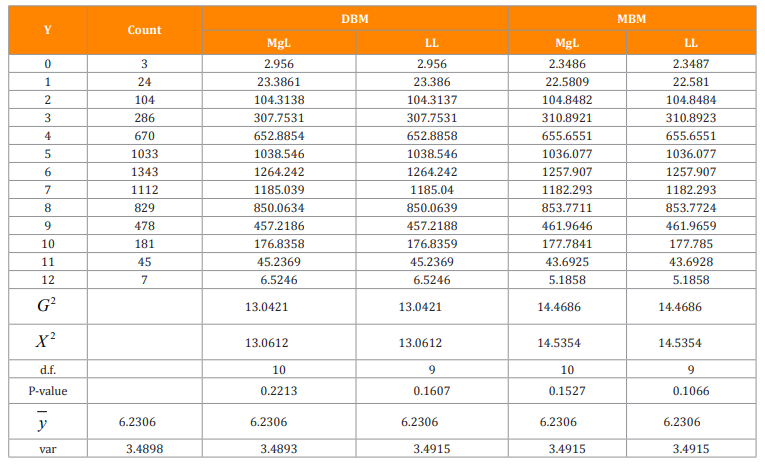Table 4: Expected values under the two models and Approaches with corresponding Pearson’s $X^{2}$ Statistic values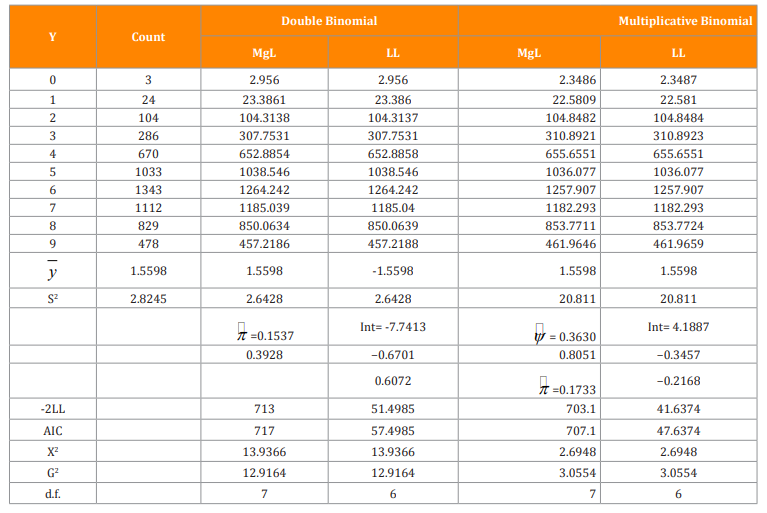Table 5: Expected Values under the two models and approaches with corresponding Pearson’s $X^{2}$ Statistic Values

### Regression Model Formulations

When there are covariates in our data, the sufficient statistic approach-here into referred to as Log-linear (LL) does not lend itself to easier formulation and implementation. Lindsey and Altham  employed this approach to fitting amongst others, the two models considered in this study to the distribution of males in families in Saxony during 1885-1976 (the human sex ratio data). This approach employs far too many parameters, 13 to be precise when the same group of models can be implemented with only four parameters with the same results. Further, the implementations under this approach are not readily available. Thus in this study, we will employ the alternative MgL procedure that utilizes PROC NLMIXED in SAS. One advantage of this is that it will based on more degrees of freedom than the log-linear model. We see for the frequency data in Tables 1 for instance, that the LL approach is based on 1 d.f. more than the MgL model based.

Example I: Teratology-Ossification on the Phalanges

Teratology is the study of abnormalities of physiological development. The offspring of animals that were exposed to a toxin during pregnancy are studied for malformation. The number of malformed offspring in a litter of size n is not typically distributed binomial because the responses of the offspring from the same litter are not independent, hence their sum does not constitute a binomial r.v. Thus, data in teratological studies exhibit over-dispersion because of the correlation among responses from off springs in the same litter.

 report data from a completely randomized design that studies the teratogenicity of phenytoin in 81 pregnant mice. The treatment structure of the experiment is an augmented factorial. In addition to an untreated control, mice received 60 mg/kg of phenytoin (PHT), 100 mg/kg of trichloropropene oxide (TCPO), and their combination. The design was augmented with a control group that was treated with water. As in , the two control groups are combined here into a single group. The presence or absence of ossification in the phalanges on both the right and left forepaws on each of the fetuses is considered a measure of the teratogenic effect. The data is presented below. For the control for instance, there are 35 pair of observations designated as (n , r). Thus, the numbers of rats in each group are respectively {35,19,16,11}.

control 35 8 8 9 9 7 9 0 5 3 3 5 8 9 10 5 8 5 8 1 6 0 5

8 8 9 10 5 5 4 7 9 10 6 6 3 5 8 9 7 10 10 10

1 6 6 6 1 9 8 9 6 7 5 5 7 9 2 5 5 6 2 8 1 8

0 2 7 8 5 7

PHT  19 1 9 4 9 3 7 4 7 0 7 0 4 1 8 1 7 2 7 2 8 1 7

0 2 3 10 3 7 2 7 0 8 0 8 1 10 1 1

TCPO  16 0 5 7 10 4 4 8 11 6 10 6 9 3 4 2 8 0 6 0 9

3 6 2 9 7 9 1 10 8 8 6 9

PHT2   11 2 2 0 7 1 8 7 8 0 10 0 4 0 6 0 7 6 6 1 6 1 7

Suppose Yij denote the number of deaths in litter i. Further, let pij be the probability of a fetus in litter i dying. Yij has the overdispersed binomial distribution with mean ni pij and variance ni pij(1 − pij )φ , with φ characterizing the correlation between any two fetuses within the same litter.

The probability of fetal death is modeled with the logit link viz: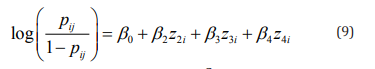We have assumed here that β0 is similar across litters, and that,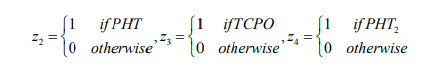Thus, the ‘control’ treatment is the reference category in this set up. Our analysis begins by fitting three different models to the data. These models are described briefly below:
• The model that assumes $p_{0}=p_{1}=p_{2}=p_{3}$ with a common dispersion parameter $\phi$ or $\omega$ for the double binomial and the multiplicative models respectively, and, where $p_{0}$ and $p_{1}$, $p_{3}$, $p_{3}$ refer respectively to the corresponding probabilities in the control and other treatment groups.
•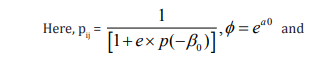• The model here has pi = pj i i ≠ j and the dispersion parameters are functions of the covariates. That is, φ = exp (a0 + a2 z2 + a3 z3 + a4 z4 ) and ω = exp(c0 +c2 z2 + c3 z3 + c4 z4 ).
• The model here has pi ≠ pj with the ps modeled as in (9) and the dispersion parameters are modeled as functions of the covariates as in the preceding case.

Results

From the results in Table 6, the two cases (II & III) with variable dispersion parameters fit better than the model in case I, where the dispersion is uniform across the four groups. Of the models in Cases II and III, the models in case III fits much better than those in case II. Case II models assume that the four groups have a common estimated probability $\pi$, which are estimated respectively as 0.2125 and 0.2158 in both the DBM and MBM. However, the models in III which assume heterogeneous success probabilities across the four groups and variable dispersion parameters (that are functions of the covariates) fit better than those in case II. The DBM here is based on $X^{2}$ = 115.1333 on 72 d.f. The estimated $\pi$ s under the MBM are functions of n, hence these values are different for different n in the final model (Case III). We may note here that the Ψs should not be mistaken for the success probabilities.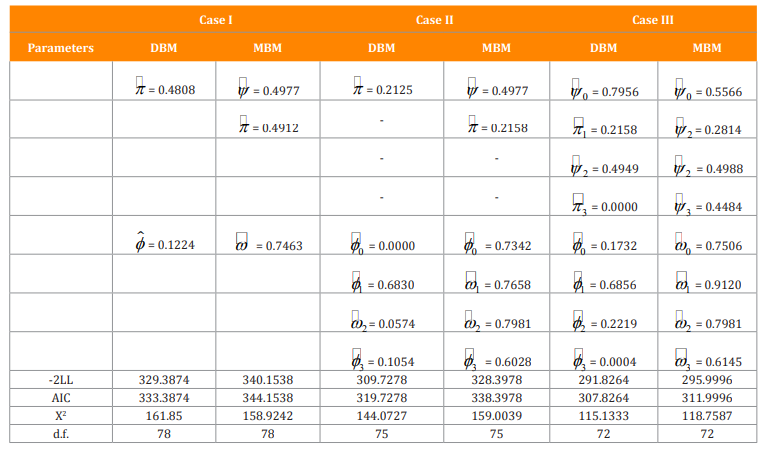Table 6: Parameter estimates for the Models in all the Cases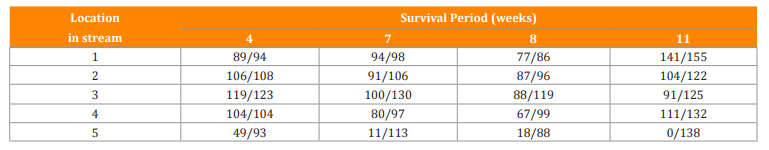Table 7: Number of Surviving eggs against number of eggs in a box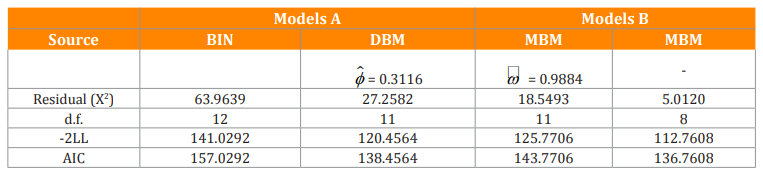Table 8: Results of Analysis of Data in Table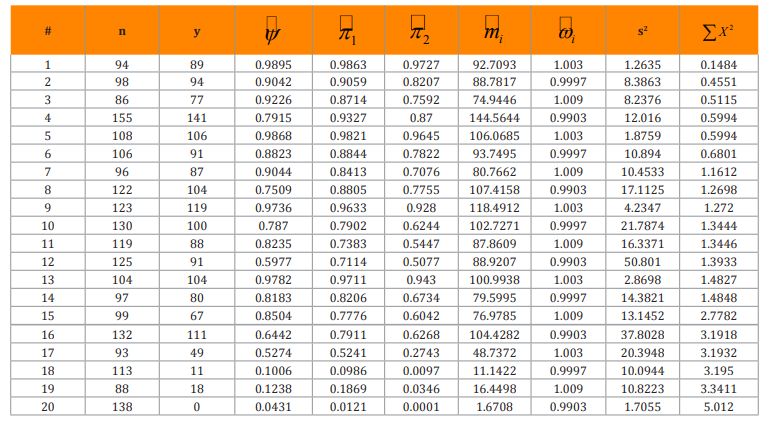Table 9: Parameter estimates under the multiplicative model with variable dispersion parameter